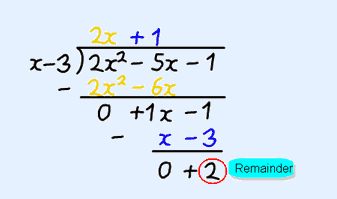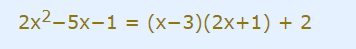Mathematics

# How to factorize a polynomial? Part-1

517 views

29 December  2020

## Introduction - Why learn The Factoring of Polynomials?

x has always been a mystery! And factoring comes to our rescue for solving problems in which one needs to find the value of x. We divide things into equal pieces. We exchange money, compare prices, understand the time, and make calculations during travel. All this often needs the skill of factoring. Apart from these, there are Geometric and scientific applications of factoring of polynomials. People use polynomials in their heads every day like in a supermarket and factorize them without even realizing it. Others, like an engineer designing a roller coaster, do it consciously.

This blog discusses the basics of polynomials, and covers 2 methods of factorization. You can find the other 3 methods in Factrorization of Polynomials - 2

## What is a Polynomial?

When we talk about factoring polynomials, we must first understand both the terms: factors and polynomials.

A polynomial can be defined as an algebraic expression composed of variables or intermediates with coefficients and constants. Exponents of the variables cannot be negative, or radicals and variables cannot be in the denominator.

For example: 9x12 + 3x3y15 – 1892z + 8, p5 + p3 – p2 +1, 96q123, 10m – 9, -5 are all polynomials.

$$\sqrt{5x^4}-\frac{7}{12}x^2+\frac{1}{\sqrt{8}}x-5\sqrt{113}$$ is also a polynomial.

But $$3x + 8x^{-5} – 3,\;\frac{2}{x}+x^3-2$$ or $$9\sqrt{x} + 4p^2$$ are not polynomials.

## Types of Polynomials:

Based on the number of terms in a polynomial, they may be classified into:

Monomials (with a single term like 4x2, 8, -9x5),

Binomials (with two terms as 3x - 9, 45x5 + 42yz),

Trinomials (with three terms) and so on.

A Linear polynomial is apolynomial with a degree* 1.

For example, 9x - 28

(*The Degree is the highest degree or exponent value of any of the terms of the polynomial or the highest sum of the exponents of all the variables in any of the terms of a polynomial. For example, the degree of polynomial 5x3 + x2y5 is 7. The degree of 5x3 is 3 while the degree of the term x2y5, 2 + 5 = 7; so the highest degree amongst all the terms is 7).

A Quadratic Polynomial refers to a polynomial with a degree 2. For example, 4x2 -3xy +5. Here the degree of 4x2 is 2; the degree of 3xy is also 2.

A Cubic Polynomial is the one with a degree 3. For example, p3 + m2 + x + 2

## Terminology:

A Factor in the simplest terms is a number or expression that divides the given number or expression entirely by leaving a remainder zero.

So, when a polynomial is written as a product of polynomials, each of the polynomials is a FACTOR of the original polynomial.

This implies that Factoring is the name given to the process of writing a polynomial as a product of polynomials.

Understanding of another term would be needed to proceed with the factorization and that is GCF or HCF.

GCF or the Greatest Common Factor is the largest quantity or term that is a factor of all the integers or polynomials involved.

The steps involved in finding the GCF of a given list of integers or polynomial terms are:

1. Prime factorize all the numbers
2. Identify the common prime factors
3. Take the product of all common prime factors
4. If there are no common prime factors, then the GCF is 1

For example:

1. To find the GCF of 12 and 8:

We know that 12= 2 × 2 × 3, and

8 = 2 × 2 × 2,

Hence the GCF (12,8) = 2 × 2 =4

2. To find the GCF of x3 and x19:

We know that x3= x . x . x , and

X19= x.x.x.x.x.x.x.x.x.x.x.x.x.x.x.x.x.x.x

Hence the GCF (x3, x19) = x . x . x = x3

3. Similarly, GCF (a3b2, a2b6 and a4b9) = a2b2

## Factoring a Polynomial

You will come across different kinds of questions like:

• Factoring cubic polynomials
• Factoring binomials
• Factoring trinomials

And maybe some others as well.### Factoring simply changes a sum into a product.

A polynomial may be factorized using various techniques like:

1. By Factoring out the HCF or the GCF
2. By using the Factor Theorem
3. By applying the re-grouping technique
4. By perfect square trinomial method
5. By the difference of the Squares method.

## Factorizing a Polynomial by Factoring out the HCF or GCF

Step 1: Find the GCF of all its terms.

Step 2: Write the polynomial as a product by FACTORING OUT the GCF from all the given terms.

Step 3: The remaining factors in each term then will form a polynomial.

For example:

\begin{align}&9x^3 – 6x + 12 x^2\\\\&= 3.x.3.x^2 – 3.x.2 + 3.x.4.x\\\\&= 3x (3x^2-2+4x)\end{align}

IMPORTANT: Remember that factoring out the GCF from a polynomial term should always be the first step in factoring a polynomial. This will then usually be followed by additional steps in the process.

## Factorizing a Polynomial by Factor Theorem

For understanding the Factor Theorem, we would first have to understand the Remainder Theorem.

We very well know that:This implies that dividing a polynomial by another polynomial gives us a remainder which may or may not be a zero. So, if we divide 2x2 – 5x -1 by x-3 using the long division method, we get 2x+1 as quotient and 2 as a remainder.Now, we can write this in an equation form as:Hence, when we divide a polynomial f(x) by a simple polynomial x-C, where C is a constant, we get a quotient q(x) and a remainder r(x).

Remember that degree of remainder r(x) is always less than the degree of divisor d(x).

So, f(x)=(x-C). q(x) + r(x)

x-C is degree 1, therefore, r(x) should be degree 0 which implies that its just another constant R

Hence, f(x) = (x-C). q(x) + R

For the value x=C, f (x)= f(C)= (C-C). q(x) + R = 0+ R = R

This indicates clearly that when we divide a polynomial by f(x) by (x-C), the remainder is F(C) and this fact is termed as the Remainder Theorem.

So, to find the remainder after dividing a polynomial by x-C, we don’t need to do any division, just calculate f(c).

## Factor Theorem is just an extension of this Remainder Theorem

Applying the Remainder theorem, and finding out the case where the remainder or f(c)=0, will give us the x-C as the factor of that polynomial as the remainder is a Zero.

So, The Factor Theorem states that when f(c) = 0, then x-C is a factor of f(x).

In other words, when x - C is a factor of f(x), then f(c)=0.

 Example:

Now Let’s apply this to find the factors of a polynomial, say: m3-13m-12.

So, f(m) = m3-13m-12

Putting the value of m as -1 we get: f (-1) = -1 + 13 - 12 =0.

Hence according to factor theorem,

[m- (-1)] or (m+1) is a factor of polynomial, m3-13m-12

Therefore, f(m) = (m + 1) (m2 - m - 12)

Also, f(m) for m = -3 => f (-3) = (-3 + 1) (9 - (-3) - 12) => (-2) (0) =0

Again, f(m) for m = 4 => f (4) = (4+1) (16-4-12) => 5 x 0 =0

Concluding from the above, f(m)= (m+1) (m+3) (m-4)

Hence, the factors of the polynomial m3-13m-12 are (m+1), (m+3), (m-4).

In other words, m3-13m-12 = (m+1) (m+3) (m-4)

## Summary

The process involved in breaking a polynomial into its factors is known as the factoring of polynomials. There are 5 methods by which we can factor a given polynomial, 2 of which have been discussed at length above with examples.

The other 3 methods have been discussed in Factorization of Polynomials-2. Hope this article makes the factoring of polynomials simpler by following the stepwise approach.

Written by Gurpreet Khatter, Cuemath Teacher

Cuemath, a student-friendly mathematics and coding platform, conducts regular Online Live Classes for academics and skill-development, and their Mental Math App, on both iOS and Android, is a one-stop solution for kids to develop multiple skills. Understand the Cuemath Fee structure and sign up for a free trial.

## What is factor theorem?

According to factor theorem, (x - a) is a factor of a polynomial p(x) if p(a) = 0.

The same statement is applicable the other way around.

If p(a) = 0, we can say that (x - a) is a factor of p(x)

## What is remainder theorem or polynomial remainder theorem?

The remainder theorem or polynomial remainder theorem states that, if a polynomial p(x) is divided by p(x - a) then the remainder is p(a).

Hence, the remainder can be found by just substituting ‘a’ in the polynomial.

p(a) will be the remainder

## What is a trinomial?

Based on the number of terms in a polynomial, they may be classified into different types of polynomials.

A polynomial with 3 terms is called a trinomial.

E.g. 3x + 4y + 7, 8x3 - 12x + 1

## What is a binomial?

Based on the number of terms in a polynomial, they may be classified into different types of polynomials.

A polynomial with 2 terms is called a binomial.

E.g. 2x + 5, 5x3 + 7x2

## What is a monomial?

Based on the number of terms in a polynomial, they may be classified into different types of polynomials.

A polynomial with 1 term is called a monomial.

E.g. x19, 5

## External References

GIVE YOUR CHILD THE CUEMATH EDGE
Access Personalised Math learning through interactive worksheets, gamified concepts and grade-wise courses